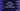# Python program to find the nth number which is square and cube of another number## Python program to find the nth number which is square and cube of another numbers:

In this post, we will learn how to find the nth number which is both square and cube of other numbers. For example, 64 is such number. It is square of 8 and cube of 4.

The first number in this series is 1, second number is 64, third number is 729, fourth number is 4096 etc.

### Algorithm to find that value:

We can use a simple algorithm to find the nth number. It is n^6 or n * n * n * n * n * n, where n is the position or nth value. This always gives one number which is square and cube of another number.

### Python program:

Let me write down this in Python:

``````n = int(input('Enter the value of n: '))

value = n * n * n * n * n * n

print('nth number which is square and cube of another numbers: {}'.format(value))``````

Here,

• We are taking the value of n and storing it in n variable.
• The value is the required value that we are calculating by multiplying n 6 times.

It will print output as like below:

``````Enter the value of n:5
nth number which is square and cube of another numbers: 15625

Enter the value of n: 4
nth number which is square and cube of another numbers: 4096``````

### Python program by using pow():

Instead of multiplying n for 6 times, we can also use pow() method. The first argument of this method is n and second argument is 6. Below is the complete program:

``````n = int(input('Enter the value of n: '))

value = pow(n, 6)

print('nth number which is square and cube of another numbers: {}'.format(value))``````

It will print similar output.

We can also print all numbers starting from 1 to n, which are square and cube of another numbers as like below:

``````n = int(input('Enter the value of n: '))

for i in range(1, n+1):
print('{}'.format(pow(i, 6)))``````

This program is taking the value of n as input from the user and it prints all n numbers which is square and cube of another numbers.

This will print output as like below:

``````Enter the value of n: 5
1
64
729
4096
15625

Enter the value of n: 10
1
64
729
4096
15625
46656
117649
262144
531441
1000000``````